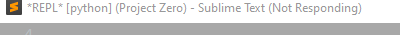# ST3 not responding while running a small mnist model

#1

Press F5 to run a simple keras mnist model, then ST3 not respond.
But the same code can be ran in vs code normally.I think it is python instead of ST that should stuck while running model.

``````model = k.Sequential([
k.layers.Flatten(input_shape=(28, 28)),
k.layers.Dense(500, activation=tf.nn.relu,),
k.layers.Dense(100, activation=tf.nn.relu,),
k.layers.Dense(10, activation=tf.nn.softmax,)])
``````
0 Likes